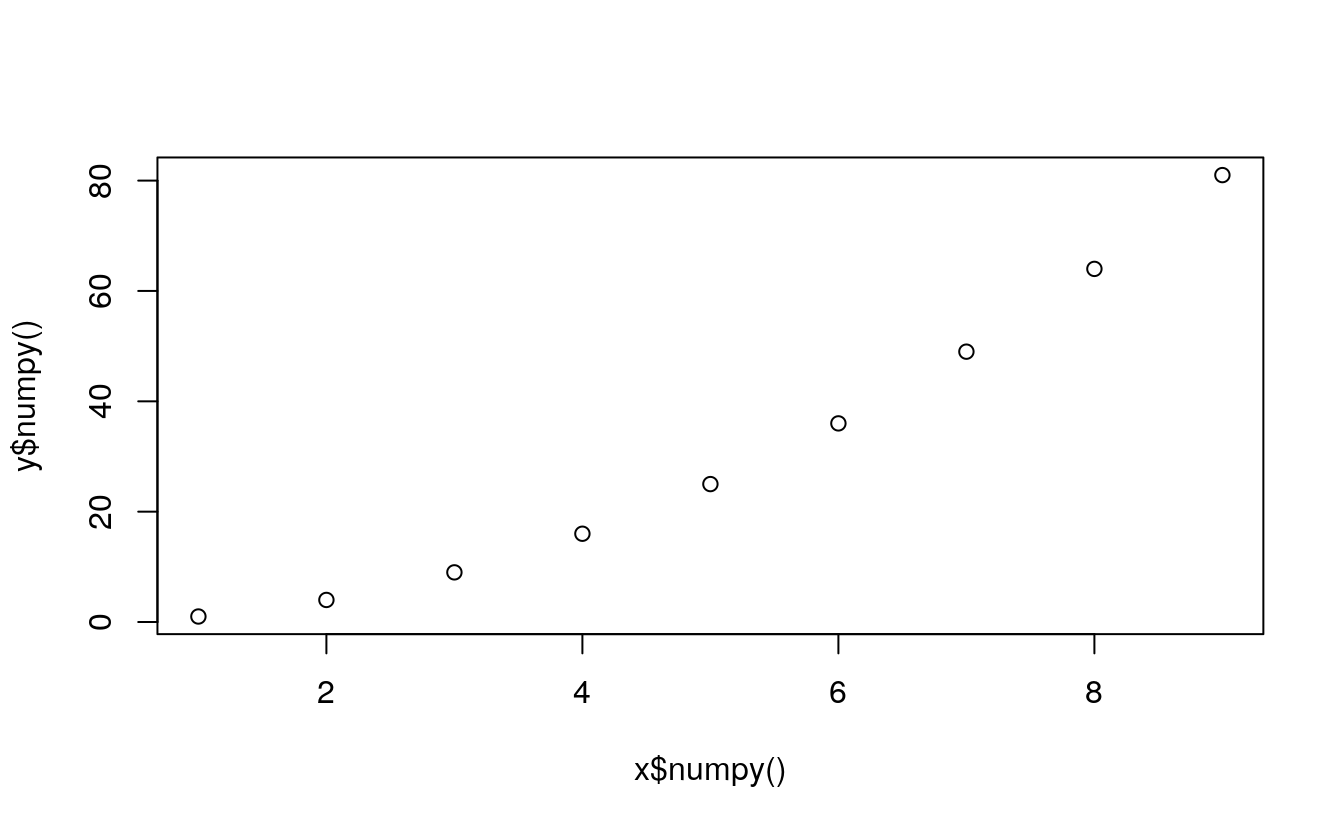# Chapter 4 Converting tensors

Last update: Sun Oct 25 13:00:09 2020 -0500 (f5e8a1973)

library(rTorch)

## 4.1 Tensor to numpy array

This is a frequent operation. I have found that this is necessary when:

• a numpy function is not implemented in PyTorch
• We need to convert a tensor to R
• Perform a boolean operation that is not directly available in PyTorch
x <- torch$arange(1, 10) y <- x^2 If we attempt to plot these two tensors we get an error: plot(x, y) #> Error in as.double(x): cannot coerce type 'environment' to vector of type 'double' They need to be converted to numpy, and then to R (which happens in the background): plot(x$numpy(), y$numpy())## 4.2numpy array to tensor • Explain how transform a tensor back and forth to numpy. • Why is this important? • In what cases in this necessary? p <- np$arange(1, 10)
class(p)
#>  "array"
(pt <- torch\$as_tensor(p))
#> tensor([1., 2., 3., 4., 5., 6., 7., 8., 9.], dtype=torch.float64)
class(pt)
#>  "torch.Tensor"          "torch._C._TensorBase"  "python.builtin.object"

### 4.2.1numpy array to R

This is mainly required for these reasons:

1. Create a data structure in R
2. Plot using r-base or ggplot2
3. Perform an analysis on parts of a tensor
4. Use R statistical functions that are not available in PyTorch

## 4.3 R objects to numpy objects

Given the R matrix $$m$$:

m <- matrix(seq(1,10), nrow = 2)
m
#>      [,1] [,2] [,3] [,4] [,5]
#> [1,]    1    3    5    7    9
#> [2,]    2    4    6    8   10

We explicitly convert it to a numpy object with the function r_to_py():

(mp <- r_to_py(m))
#> [[ 1  3  5  7  9]
#>  [ 2  4  6  8 10]]
class(mp)
#>  "numpy.ndarray"         "python.builtin.object"
class(mp)
#>  "numpy.ndarray"         "python.builtin.object"